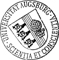## Numerical Simulation of Surface Acoustic Wave Actuated Cell Sorting

• We consider the mathematical modeling and numerical simulation of high throughput sorting of two different types of biological cells (type I and type II) by a biomedical micro-electro-mechanical system (BioMEMS) whose operating behavior relies on surface acoustic wave (SAW) manipulated fluid flow in a microchannel. The BioMEMS consists of a separation channel with three inflow channels for injection of the carrier fluid and the cells, two outflow channels for separation, and an interdigital transducer (IDT) close to the lateral wall of the separation channel for generation of the SAWs. The cells can be distinguished by fluorescence. The inflow velocities are tuned such that without SAW actuation a cell of type I leaves the device through a designated outflow channel. However, if a cell of type II is detected, the IDT is switched on and the SAWs modify the fluid flow such that the cell leaves the separation channel through the other outflow boundary. The motion of a cell in the carrierWe consider the mathematical modeling and numerical simulation of high throughput sorting of two different types of biological cells (type I and type II) by a biomedical micro-electro-mechanical system (BioMEMS) whose operating behavior relies on surface acoustic wave (SAW) manipulated fluid flow in a microchannel. The BioMEMS consists of a separation channel with three inflow channels for injection of the carrier fluid and the cells, two outflow channels for separation, and an interdigital transducer (IDT) close to the lateral wall of the separation channel for generation of the SAWs. The cells can be distinguished by fluorescence. The inflow velocities are tuned such that without SAW actuation a cell of type I leaves the device through a designated outflow channel. However, if a cell of type II is detected, the IDT is switched on and the SAWs modify the fluid flow such that the cell leaves the separation channel through the other outflow boundary. The motion of a cell in the carrier fluid is modeled by the Finite Element Immersed Boundary Method (FE-IB) featuring a coupled system consisting of the incompressible Navier-Stokes equations with respect to a Cartesian coordinate system and the equation of motion of the cell described in a Lagrangian framework. The generation of the SAWs is taken care of by the linearized equations of piezoelectricity, and the impact of the SAWs on the fluid flow is realized by means of a boundary condition for the Navier-Stokes equations. The discretization in space is done by P2/P1 Taylor-Hood elements for the fluid flow and periodic cubic splines for the immersed cell, whereas for discretization in time we use the backward Euler scheme for the Navier-Stokes equations and the forward Euler scheme for the equation of motion of the immersed cell. This backward Euler/forward Euler Finite Element Immersed Boundary Method (BE/FE FE-IB) requires a CFL-type condition for stability. Numerical results are presented that illustrate the feasibility of the surface acoustic wave actuated cell sorting approach.Author: Thomas FrankeGND, Ronald H. W. HoppeGND, Christopher LinsenmannGND, Kidist Zeleke urn:nbn:de:bvb:384-opus4-18775 https://opus.bibliothek.uni-augsburg.de/opus4/1877 Preprints des Instituts für Mathematik der Universität Augsburg (2012-06) Preprint English Universität Augsburg 2012/05/02 surface acoustic wave actuated cell sorting; biomedical micro-electro-mechanical-systems; finite element immersed boundary method Finite-Elemente-Methode; MEMS; Akustische Oberflächenwelle; Zellfraktionierung; Lab on a Chip Mathematisch-Naturwissenschaftlich-Technische Fakultät Mathematisch-Naturwissenschaftlich-Technische Fakultät / Institut für Mathematik Mathematisch-Naturwissenschaftlich-Technische Fakultät / Institut für Mathematik / Lehrstuhl für Numerische Mathematik 5 Naturwissenschaften und Mathematik / 51 Mathematik / 510 MathematikDeutsches Urheberrecht mit Print on Demand